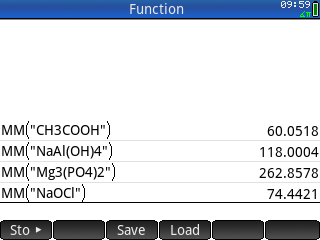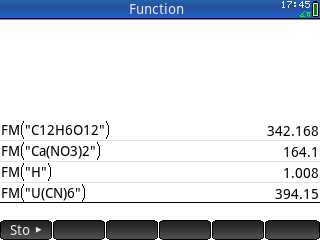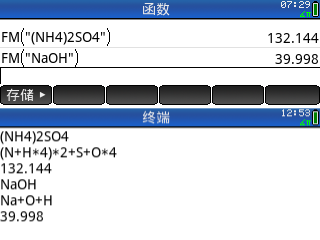## Erik Swanson

ID:2549
First Name:Erik
Last Name:Swanson
Last Change:2021-12-31
Number of Files:3 (544th most prolific)
 Calculate Molar Mass of Compound 1.0   (details) Prime ENG   201KB/7KBSimple command-line program to determine the molar mass (formula mass, molecular weight) of a compound. It is a reworking of %8779%. By Aaron Kennedy and Erik Swanson. 2021/12/31
 Formula Mass of a Compound   (details) Prime ENG   2KB/8KBThis program finds the formula mass (in grams/mole) of any chemical compound typed into the calculator using standard symbolic notation for elements (e.g. "H", "Ca", "O", etc.). By Erik Swanson. 2018/02/18
 Formula Mass of a Compound 2.0   (details) Prime ENG   27KB/7KBThis program finds the formula mass (in grams/mole) of any chemical compound typed into the calculator using standard symbolic notation for elements (e.g. "H", "Ca", "O", etc.). This improved version fixes some serious problems in the old version (Formula Mass of a Compound). By Erik Swanson and Yang Xiao (肖扬). 2021/12/31